# 1.6 Code Samples

The code samples in this book are written in pseudocode. These should be easy enough to read for anyone who has any programming experience in any of the most common programming languages of the last 40 years. To get an idea of what the pseudocode in this book looks like, here is a function that computes the average of an array,: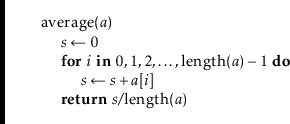As this code illustrates, assigning to a variable is done using thenotation. We use the convention that the length of an array,, is denoted by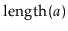and array indices start at zero, so thatare the valid indices for. To shorten code, and sometimes make it easier to read, our pseudocode allows for (sub)array assignments. The following two functions are equivalent: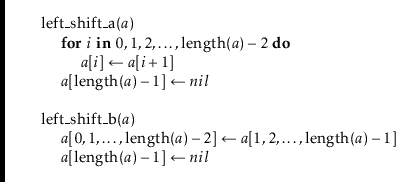The following code sets all the values in an array to zero: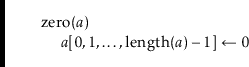When analyzing the running time of code like this, we have to be careful; statements likeordo not run in constant time. They run intime.

We take similar shortcuts with variable assignments, so that the codesets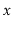to zero andto 1 and the codeswaps the values of the variablesand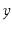.

Our pseudocode uses a few operators that may be unfamiliar. As is standard in mathematics, (normal) division is denoted by the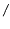operator. In many cases, we want to do integer division instead, in which case we use theoperator, so thatis the integer part of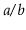. So, for example,but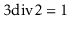. Occasionally, we also use the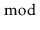operator to obtain the remainder from integer division, but this will be defined when it is used. Later in the book, we may use some bitwise operators including left-shift (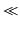), right shift (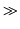), bitwise and (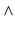), and bitwise exclusive-or ().

The pseudocode samples in this book are machine translations of Python code that can be downloaded from the book's website.1.2 If you ever encounter an ambiguity in the pseudocode that you can't resolve yourself, then you can always refer to the corresponding Python code. If you don't read Python, the code is also available in Java and C++. If you can't decipher the pseudocode, or read Python, C++, or Java, then you may not be ready for this book.

#### Footnotes

... website.1.2
http://opendatastructures.org
opendatastructures.org